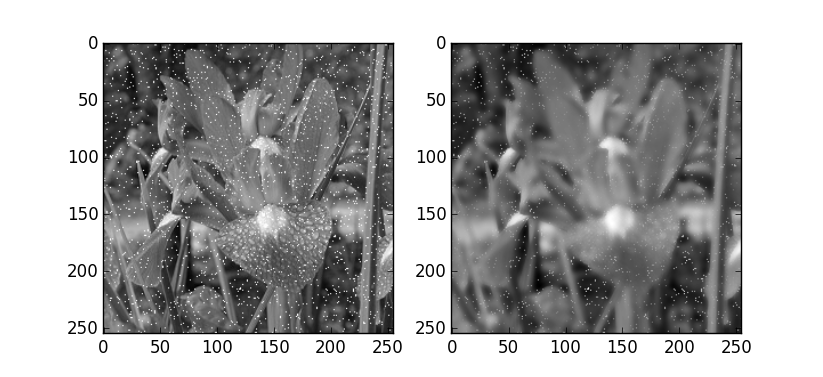# Python下opencv使用笔记（五）（图像的平滑与滤波）

## （一）统一的2D滤波器cv2.filter2D

Opencv提供的一个通用的2D滤波函数为cv2.filter2D()，滤波函数的使用需要一个核模板，对图像的滤波操作过程为：将和模板放在图像的一个像素A上，求与之对应的图像上的每个像素点的和，核不同，得到的结果不同，而滤波的使用核心也是对于这个核模板的使用，需要注意的是，该滤波函数是单通道运算的，也就是说对于彩色图像的滤波，需要将彩色图像的各个通道提取出来，对各个通道分别滤波才行。

import cv2
import numpy as np
import matplotlib.pyplot as plt

img = cv2.imread('flower.jpg',0) #直接读为灰度图像
img1 = np.float32(img) #转化数值类型
kernel = np.ones((5,5),np.float32)/25

dst = cv2.filter2D(img1,-1,kernel)
#cv2.filter2D(src,dst,kernel,auchor=(-1,-1))函数：
#输出图像与输入图像大小相同
#中间的数为-1，输出数值格式的相同plt.figure()
plt.subplot(1,2,1),plt.imshow(img1,'gray')#默认彩色，另一种彩色bgr
plt.subplot(1,2,2),plt.imshow(dst,'gray')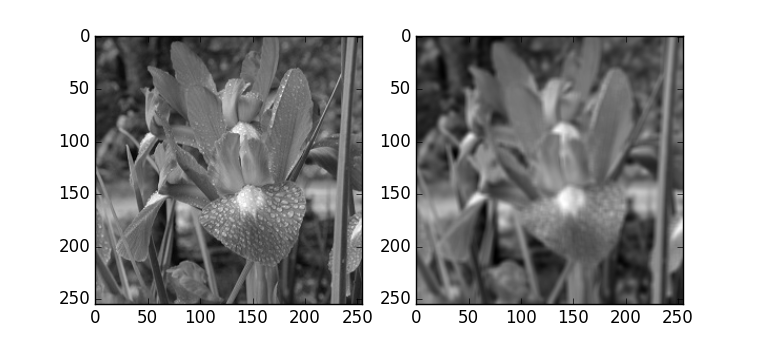## （二）均值滤波

M=19111111111

import cv2
import matplotlib.pyplot as plt
img = cv2.imread('flower.jpg',0) #直接读为灰度图像
blur = cv2.blur(img,(3,5))#模板大小3*5
plt.subplot(1,2,1),plt.imshow(img,'gray')#默认彩色，另一种彩色bgr
plt.subplot(1,2,2),plt.imshow(blur,'gray')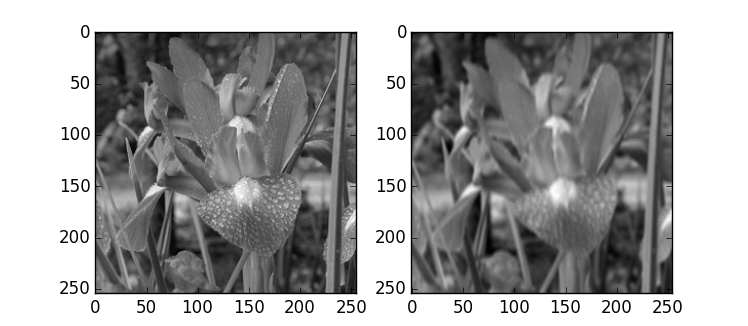## （三）高斯模糊模板

import cv2
import numpy as np
import matplotlib.pyplot as plt

img = cv2.imread('flower.jpg',0) #直接读为灰度图像
for i in range(2000): #添加点噪声
temp_x = np.random.randint(0,img.shape)
temp_y = np.random.randint(0,img.shape)
img[temp_x][temp_y] = 255
blur = cv2.GaussianBlur(img,(5,5),0)
plt.subplot(1,2,1),plt.imshow(img,'gray')#默认彩色，另一种彩色bgr
plt.subplot(1,2,2),plt.imshow(blur,'gray')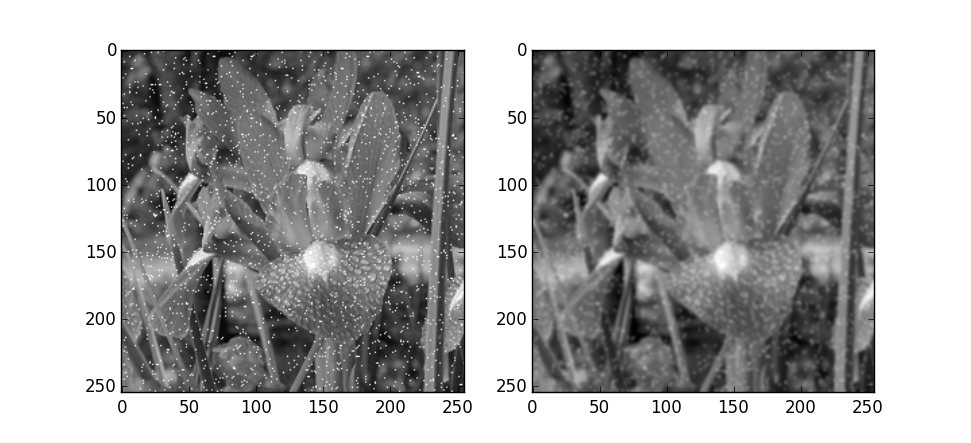## （四）中值滤波模板

import cv2
import numpy as np
import matplotlib.pyplot as plt

img = cv2.imread('flower.jpg',0) #直接读为灰度图像
for i in range(2000): #添加点噪声
temp_x = np.random.randint(0,img.shape)
temp_y = np.random.randint(0,img.shape)
img[temp_x][temp_y] = 255

blur = cv2.medianBlur(img,5)
plt.subplot(1,2,1),plt.imshow(img,'gray')#默认彩色，另一种彩色bgr
plt.subplot(1,2,2),plt.imshow(blur,'gray')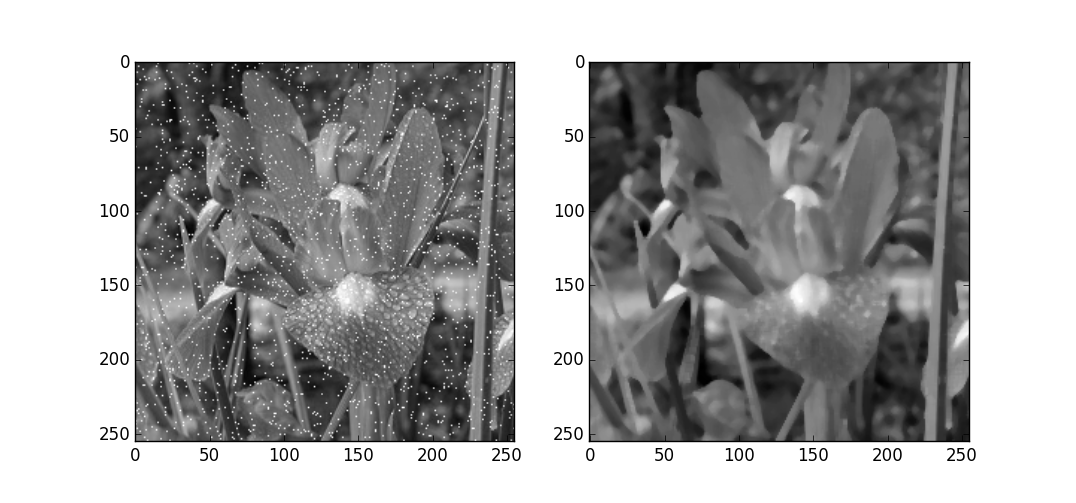## （五）双边滤波

cv2.bilateralFilter(img,d,’p1’,’p2’)函数有四个参数需要，d是领域的直径，后面两个参数是空间高斯函数标准差和灰度值相似性高斯函数标准差。一个实例如下：

import cv2
import numpy as np
import matplotlib.pyplot as plt
img = cv2.imread('flower.jpg',0) #直接读为灰度图像
for i in range(2000): #添加点噪声
temp_x = np.random.randint(0,img.shape)
temp_y = np.random.randint(0,img.shape)
img[temp_x][temp_y] = 255

#9---滤波领域直径
#后面两个数字：空间高斯函数标准差，灰度值相似性标准差
blur = cv2.bilateralFilter(img,9,75,75)
plt.subplot(1,2,1),plt.imshow(img,'gray')#默认彩色，另一种彩色bgr
plt.subplot(1,2,2),plt.imshow(blur,'gray')